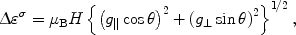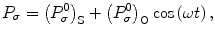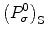# Electron-Spin Precession in Dependence of the Orientation of the External Magnetic Field

## Abstract

Electron-spin dynamics in semiconductor-based heterostructures has been investigated in oblique magnetic fields. Spins are generated optically by a circularly polarized light, and the dynamics of spins in dependence of the orientation (θ) of the magnetic field are studied. The electron-spin precession frequency, polarization amplitude, and decay rate as a function ofθ are obtained and the reasons for their dependences are discussed. From the measured data, the values of the longitudinal and transverse components of the electrong-factor are estimated and are found to be in good agreement with those obtained in earlier investigations. The possible mechanisms responsible for the observed effects are also discussed.

## Introduction

Spintronics  has been built on the spin degree of freedom and discusses the idea of using information carried by the spin of the electron in electronic devices. Recently, it has gained a lot of attention [4, 5], which may result in devices with increased capability and functionality beyond well-established storage or memory applications, already implemented as giant magnetoresistance read-heads and nonvolatile magnetic random access memory .

However, one of the important requirements necessary in developing spintronic devices is the efficient generation of spins in a semiconductor and transporting them reliably over reasonable distances and then detecting them. Much effort  has thus been spent in understanding these issues in semiconductors. Generation of spin polarization usually means creating a nonequilibrium spin population. This has been achieved either by optical methods (circularly polarized light, σ, excitation) or by magnetic semiconductors, or ferromagnetic contacts [2, 5, 7]. Several attempts, e.g., using ferromagnetic contacts to Si or InAs-based heterostructures (quantum wells, QWs), have resulted in low spin injection effects due to the “conductivity mismatch” (more precisely, a mismatch between effective resistances in the metal and in semiconductor host) or other reasons. However, the spin generation by the optical methods has been successful and the high spin-polarization of conductor band electrons in semiconductor heterostructures has been obtained . Despite substantial progress in optical spin generation, a further hurdle still remains in the spin transport is the lack of a proper understanding of spin dynamics in semiconductor-based heterostructures .

In the previous study , we focused on spin dynamics in a transverse magnetic field in GaAs QWs by circularly polarized photoluminescence (PL) measurements. We studied the bias-dependent circular polarization of PL (P σ ) and found that P σ decays in the transverse magnetic field with an enhancement of increasing the strength of the negative bias. In this study, we extend our investigation and study the electron-spin dynamics in dependence of the orientation (θ) of the magnetic field. The spin precession frequency, amplitude, and decay rate as a function of θ are estimated and their dependences on θ are discussed. The values of the longitudinal and transverse components of the electron g-factor are also estimated.

## Experimental

Investigated samples were GaAs double QWs separated by a relatively thin (~20 nm) Al0.3Ga0.7As barrier. The thickness of the QWs was varied from 8 to 10 nm. The samples were grown on the Si-doped GaAs substrate using the MBE growth technique. For the application of the negative external bias of magnitude 2.5 V normal to the heretostructure layers, the top surface of the sample was coated with a semitransparent electrode. The sample was mounted in a chip-carrier. We measured the PL excited by ps pulses of a tunable Ti:sapphire laser with a repetition rate of 76 MHz using a streak camera . All the measurements were done at liquid helium temperature by placing the chip-carrier in a temperature-regulated cryostat. The PL was excited directly to the exciton absorption band and was detected with the small long-wavelength shift to minimize the polarization losses. The exciting beam was directed perpendicular to the 5-T magnetic field direction, and the PL was detected in the backward direction. The θ with respect to the growth axis of the heterostructure was changed by rotating the chip-carrier. The degree of circular polarization P σ was calculated using the relation(1)

whereis the intensity of PL in the right(left), σ+(σ), circularly polarization under σ+ light excitation.

## Results and Discussion

The PL was measured in the right (σ+) and left (σ) circularly polarizations under σ+ light excitation in the presence of external bias and magnetic field, and Pσ was calculated from the measured data. Figure 1 shows the kinetics of Pσ, where the variations in the dynamics of Pσ with the direction θ of the magnetic field is clearly seen. When θ deviates from 90°, a non-oscillating weakly damping component arises, with its amplitude growing with the deviation angle. The amplitude of the oscillating component also decreases. An analysis of the oscillating part of the signal has been performed. The result has shown that it can be well approximated by the damping harmonic oscillating function(2)

where P σ 0 = P σ (0) is the amplitude, ω is the oscillation frequency, and τ is the oscillation decay rate. Equation 2 allowed us to calculate the dependencies ofω, and τ on θ. In Fig. 2, we plot the dependence of the oscillation frequency ω on θ, where one can see that the deviation from the exact Voigt configuration (θ = 90°) is accompanied by an increase in ω. The increase in ω upon deviation of the magnetic field from the transverse direction (θ = 90°) is related to the anisotropy of the electron g-factor resulting from the quantum size effect or quantum confined (quantization) effect . The splitting between the spin sublevels of the free electron in quasi-two-dimensional structures is given  by(3)

where μB is the Bohr magneton, H is the magnetic field, and g and g are the longitudinal and transverse components of the electron g-factor, respectively. Equation 3 is fitted to the data shown in Fig. 2 (solid line). From the fit, we obtain the values of the corresponding components for the QW structure. The values are g = 0.25 and g = 0.20, agreeing well with the values obtained in the earlier investigations .

Figure 3 shows the dependence of the initial oscillation amplitude on the angle between the magnetic field and the direction of observation. The changes in the amplitude of the oscillations in oblique magnetic fields can be explained as follows. As Pσ is determined by the orientation of the electron spin with respect to the direction of observation, optical excitation aligns the electron spin along the longitudinal () axis. When the electron-spin precesses around the transverse magnetic field, its projection onto the direction of observation periodically changes the sign, which leads to the oscillations of Pσ. In the longitudinal magnetic field (θ = 0°), the projection of the electron spin onto the direction of observation does not change with time and, therefore, Pσ remains constant. For the intermediate values of θ Pσ contains both the oscillating and smooth components :whereis the smooth component of Pσ, and the amplitudes of these components are determined by the longitudinal (H) and transverse (H) components of the magnetic field. The amplitude of the oscillatory component is(4)

where H = H cos θ and H = H sin θ. Equation 4 is plotted in Fig. 3 (solid line), where it can be seen that the theoretic curve agrees well with the experimental data.

The dependence of the oscillation decay rate on the orientation of the magnetic field is shown in Fig. 4. As can be seen, the change ofθ from 90° is accompanied by a substantial increase in the decay rate. The angular dependence on the decay of the oscillations is mainly related to the spread of the electrong-factors (gandg) as well as to the residual exchange interaction between the electron spin and rapidly relaxing spin of the hole. Sincegandgare close in magnitude, the contribution from a difference in the spread of their values reveals not to be dominating in the observed effects. As the possibility of the exchange interaction looks likely, it will be discussed briefly.

The exchange interaction is an effect of an effective magnetic field directed along the spin of the hole (parallel to the longitudinal axis, here ) acting on the electron spin. The speed and direction of the electron-spin precession are determined by the total field Htot: a vector sum of the magnetic (H) and exchange (hex) fields. The direction of hex is parallel to the growth axis of the heterostructure. The instantaneous value of the fluctuating exchange field corresponding to the exchange splitting ∆ex can be written as hex = Δex/μBg. The magnitude and direction of the exchange field are determined by the state of the hole spin, which is a linear combination of the heavy-hole band states |+3/2〉 and |−3/2〉 for the opposite spin directions . The coefficients of the combination determine the polarization states and conditions. For example, for excitation with a σ light, the coefficients take the values of 0 and 1 for the states |+3/2〉 and |−3/2〉, respectively [18, 19]. When the hole spin is relaxed, the coefficients acquire random values, and the mean value of hex vanishes, with its instantaneous values varying in time in a random way, and as a result, a fluctuating component arises in Htot acting on the electron spin. The fluctuating component broadens the frequency spectrum of the oscillations and thus accelerates the oscillations decay 1/τ, which results in an additional damping of the oscillations of the Pσ. However, the contribution of the exchange interaction into this damping depends on mutual orientation of the exchange and magnetic fields. The oscillation frequency (ω)ex is given by (ω)ex = μB{g2(hex + H cos θ)2 + g2(H sin θ)2}1/2, from which the effect of hex on the oscillation frequency can be seen as a function of the orientation of the external magnetic field H. For example, when deviating from the Voigt configuration (θ = 90°), the external magnetic field component H(=H cos θ) arises, which is linearly combined with hex and as a result, the contribution of the frequency fluctuating component to the Htot increases for an increase in 1/τ in the heterostructures.

## Conclusions

Electron-spin dynamics in GaAs-based heterostructures was investigated in the presence of a negative external bias. Electron spins were generated optically by a circularly polarized light and the dynamics of spins in dependence of the orientation of the magnetic field was studied. The spin precession frequency, amplitude, and polarization decay rate were found and their dependences on the orientation of the magnetic field were discussed. The values ofgandgwere also estimated. The mechanisms responsible for the observed effects were discussed briefly.

## References

1. 1.

Ziese M., Thornton M.J. (Eds): Spin Electronics. Springer-Verlag, Heidelberg; 2001.

2. 2.

Awschalom D.D., Loss D., Samarth N. (Eds): Semiconductor Spintronics and Quantum Computation. Springer, Berlin; 2002.

3. 3.

Dyakonov MI, Khaetskii AV: Spin hall effect. In Spin Physics in Semiconductors. Edited by: Dyakonov M.I.. Springer-Verlag, Berlin; 2008. 10.1007/978-3-540-78820-1

4. 4.

Sarma SD: Am. Sci.. 2001, 89: 516. Bibcode number [2001AmSci..89..516D] Bibcode number [2001AmSci..89..516D]

5. 5.

Miah MI: J. Optoelectron. Adv. Mater.. 2008, 10: 2487.

6. 6.

Prinz GA: Science. 1998, 282: 1660. COI number [1:CAS:528:DyaK1cXnslGhsLs%3D] 10.1126/science.282.5394.1660

7. 7.

Jia YQ, Shi RC, Chou SY: IEEE Trans. Magn.. 1996, 32: 4707. ; COI number [1:CAS:528:DyaK28XmsFWrtro%3D]; Bibcode number [1996ITM....32.4707J] 10.1109/20.539125

8. 9.

Mandl F: Quantum Mechanics. Wiley, New York; 1998.

9. 10.

Haug H (Ed): Optical Nonlinearities and Instabilities in Semiconductors. Academic Press, New York; 1988.

10. 11.

van Kesteren HW, Cosman EC, van der Poel WAJA, Foxon CT: Phys. Rev. B. 1990, 41: 5283. Bibcode number [1990PhRvB..41.5283V] Bibcode number [1990PhRvB..41.5283V] 10.1103/PhysRevB.41.5283

11. 12.

Ivchenko EL, Kiselev AA: Sov. Phys. Semicond.. 1992, 26: 827.

12. 13.

Malinowski A, Harley RT: Phys. Rev. B. 2000, 62: 2051. ; COI number [1:CAS:528:DC%2BD3cXkvVelsrc%3D]; Bibcode number [2000PhRvB..62.2051M] 10.1103/PhysRevB.62.2051

13. 14.

Ivchenko EL, Kiselev AA, Willander M: Solid State Commun.. 1997, 102: 375. ; COI number [1:CAS:528:DyaK2sXislGrtr4%3D]; Bibcode number [1997SSCom.102..375I] 10.1016/S0038-1098(97)00009-4

14. 15.

Maialle MZ, De A Silva EA, Sham LJ: Phys. Rev. B. 1993, 47: 15776. ; COI number [1:CAS:528:DyaK3sXksFyntbo%3D]; Bibcode number [1993PhRvB..4715776M] 10.1103/PhysRevB.47.15776

15. 16.

Chaichian M, Hagedorn R: Symmetries in Quantum Mechanics. Institute of Physics Publishing, London; 1998. 10.1887/0750304081

16. 17.

Kane EO: J. Phys. Chem. Solids. 1957, 1: 249. Bibcode number [1957JPCS....1..249K] Bibcode number [1957JPCS....1..249K] 10.1016/0022-3697(57)90013-6

17. 18.

Lampel G, Weisbuch C: Solid State Commun.. 1975, 16: 877. ; COI number [1:CAS:528:DyaE2MXkt1Snt7o%3D]; Bibcode number [1975SSCom..16..877L] 10.1016/0038-1098(75)90884-4

18. 19.

Pikus GE, Titkov AN: Optical Orientation, Modern Problems in Condensed Matter Science. Edited by: Meier F, Zakharchenya BP. North-Holland, Amsterdam; 1984.

## Author information

Authors

### Corresponding author

Correspondence to M Idrish Miah.

## Rights and permissions

Reprints and Permissions

Miah, M.I. Electron-Spin Precession in Dependence of the Orientation of the External Magnetic Field. Nanoscale Res Lett 4, 588 (2009). https://doi.org/10.1007/s11671-009-9283-0